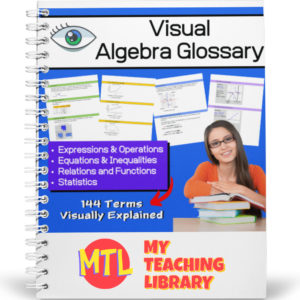Showing all 4 results

• Sale!\$7.00 \$5.50

This consumer math bundle will teach students about both simple and compound interest and give them the practice they need to help them master solving word problems!

Includes:

•\$4.00

This consumer math resource has been designed to help teach students and give them the practice they need to master the concepts taught.

The first two pages will explain interest (simple and compound) and give students the formulas they will need to solve the word problems. There are 60 word problems in all. 3 sets of 10 simple interest problems and 3 sets of 10 compound interest problems. Answer keys are provided.

•\$15.00

Give your Algebra I and Algebra II students a tool they can really use! This Visual Algebra Glossary will be an invaluable resource giving them explanations, definitions and examples of 144 terms. Easy to follow and understand, students will turn to this resource while learning new concepts, completing work and studying for exams.

There are four color coded sections, each with an term index:

• – Expressions & Operations
• – Equations & Inequalities
• – Relations & Functions
• – Statistics

73 pages

•\$4.00

This 123 page math resource will help students who are learning (or struggling to understand) algebra better by giving detailed explanations of related terminology and concepts. This book as been categorized as curriculum because of the detailed explanations of the topics. It however, is for instructional use only and does not include student problems.

(See description below for covered topics)